$$\def\RR{\bf R} \def\real{\mathbb{R}} \def\bold#1{\bf #1} \def\d{\mbox{Cord}} \def\hd{\widehat \mbox{Cord}} \DeclareMathOperator{\cov}{cov} \DeclareMathOperator{\var}{var} \DeclareMathOperator{\cor}{cor} \newcommand{\ac}{\left\{#1\right\}}$$

## Graphical Models for Brain Connectivity: Algebraic (Non-likelihood) Methods

### Xi (Rossi) Luo

Brown University
Department of Biostatistics
Center for Statistical Sciences
Computation in Brain and Mind
Brown Institute for Brain ScienceSAMSI Working Group
February 23, 2016

Funding: NSF/DMS 1557467; NIH P20GM103645, P01AA019072, P30AI042853; AHA$10^{11}$ neurons
Ex: DTI, DWI, ...
or optogenetics modeling Luo et al, 16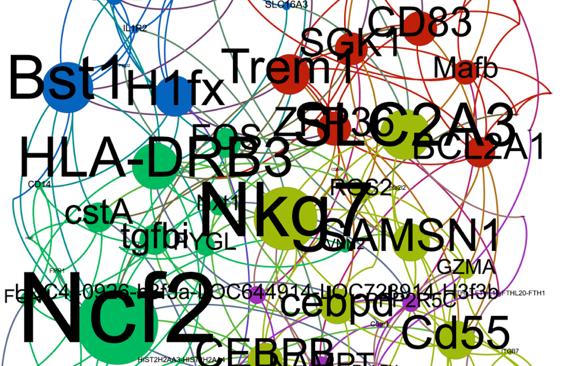$10^4$ genes, $10^6$ SNPs
Ex: Gene networks Liu & Luo, 15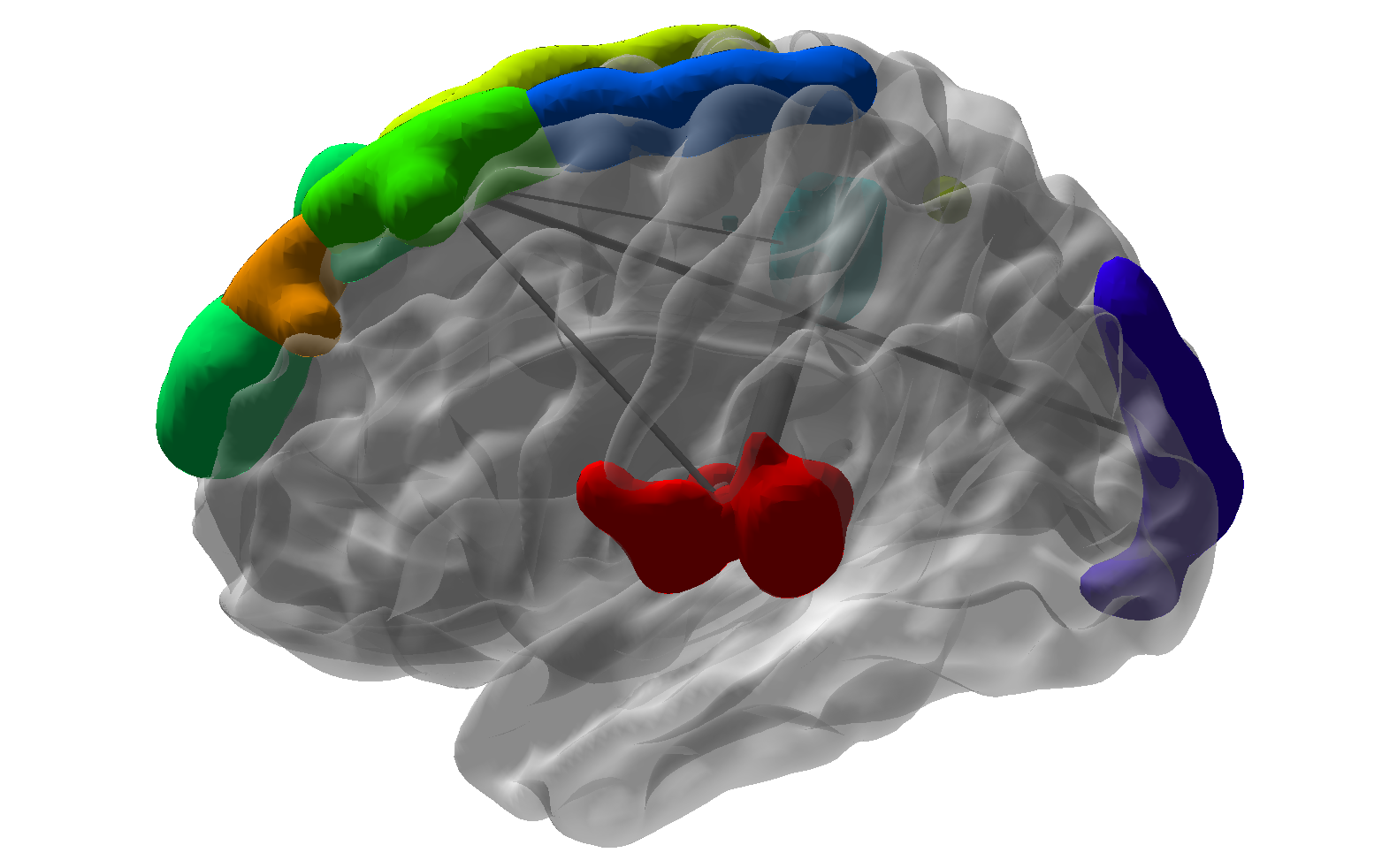$10^6$ functional MRI voxels
This Talk

## fMRI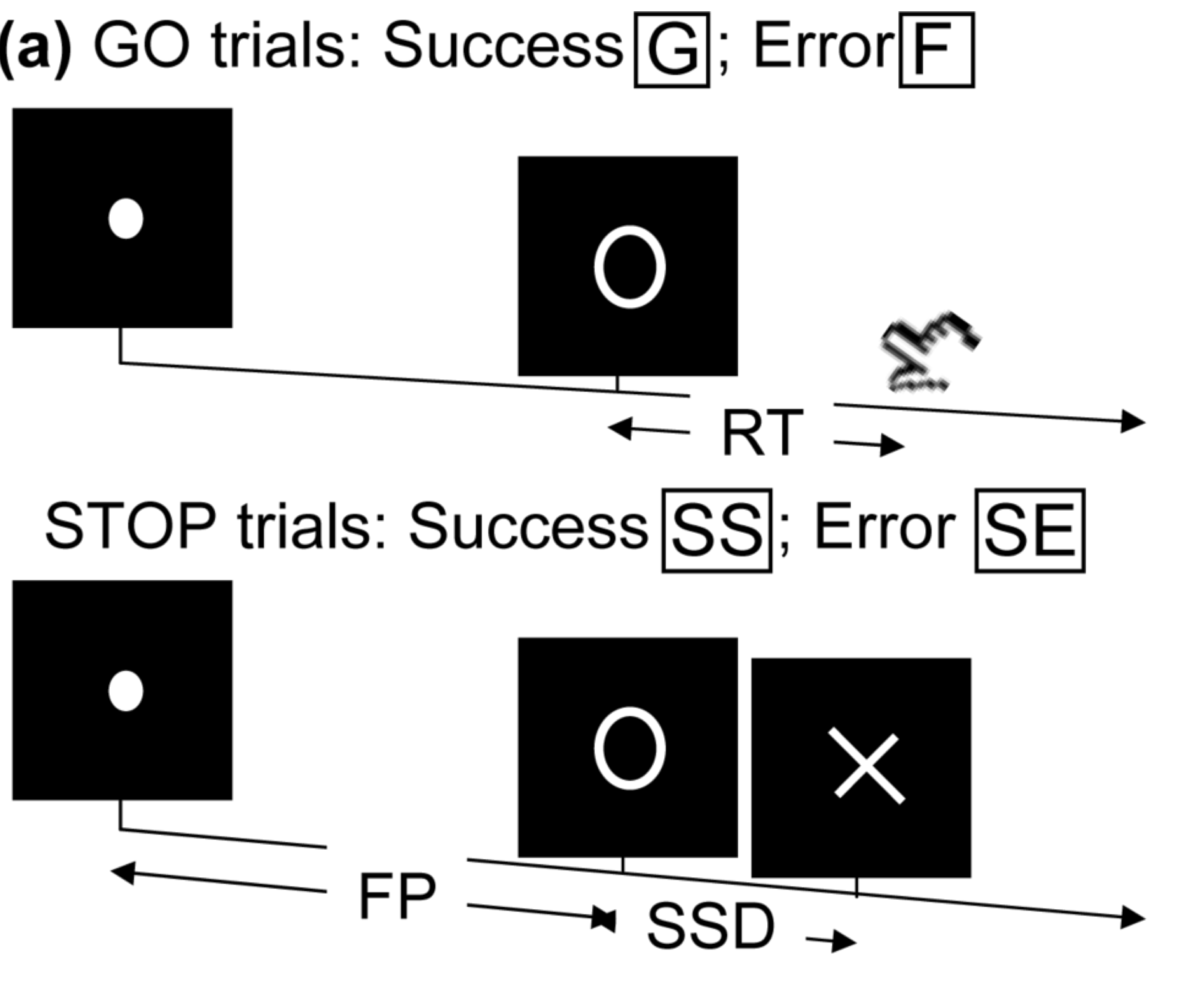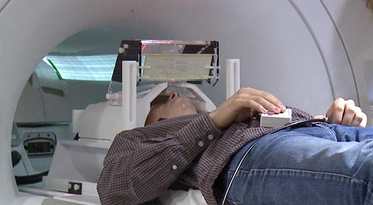• Resting-state fMRI: "rest" in scanner

This talk: resting-state fMRI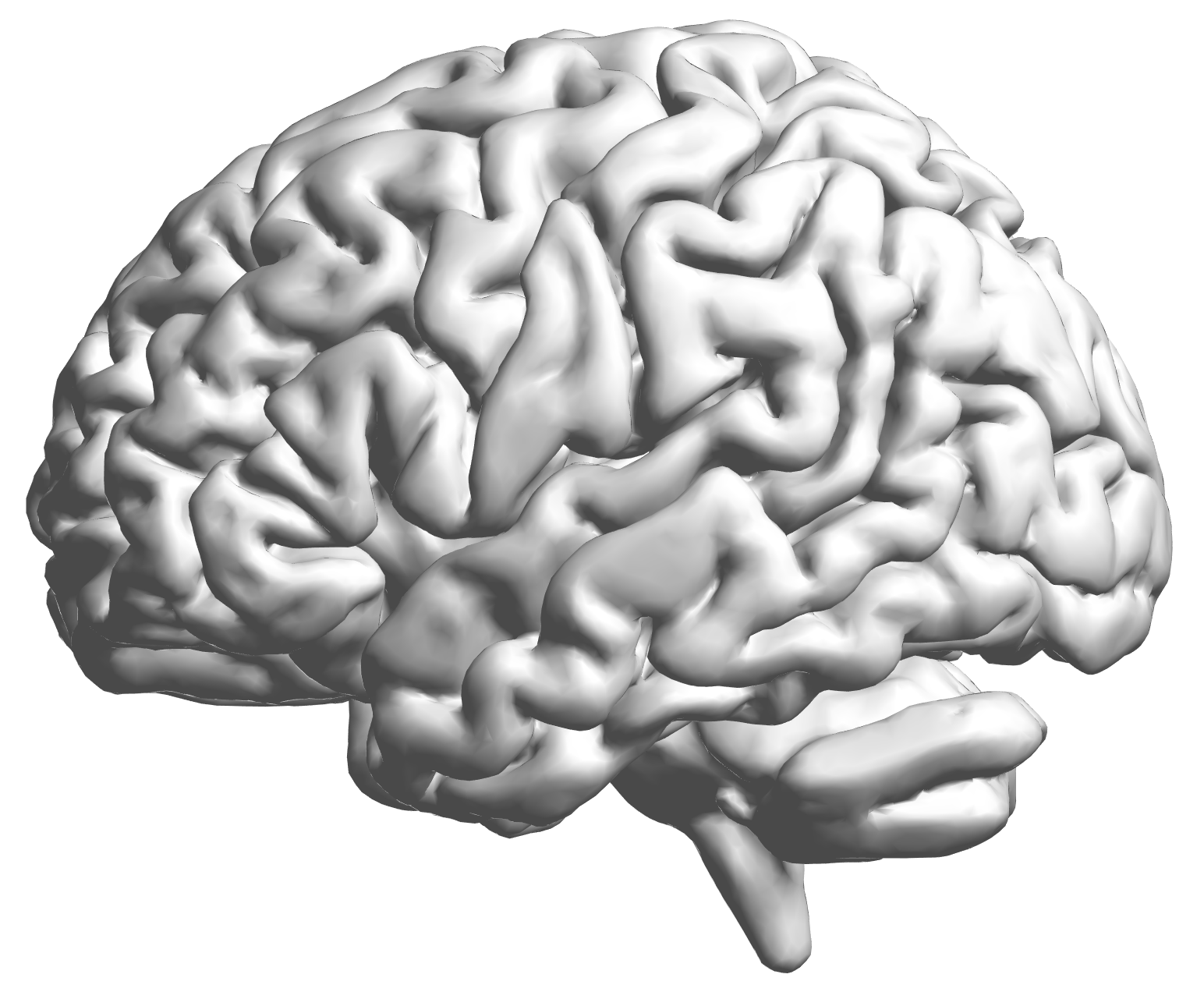fMRI data: blood-oxygen-level dependent signals from each cube/voxel (~millimeters), $10^5$ ~ $10^6$ voxels in total.

## fMRI Studies

Sub 1, Sess 1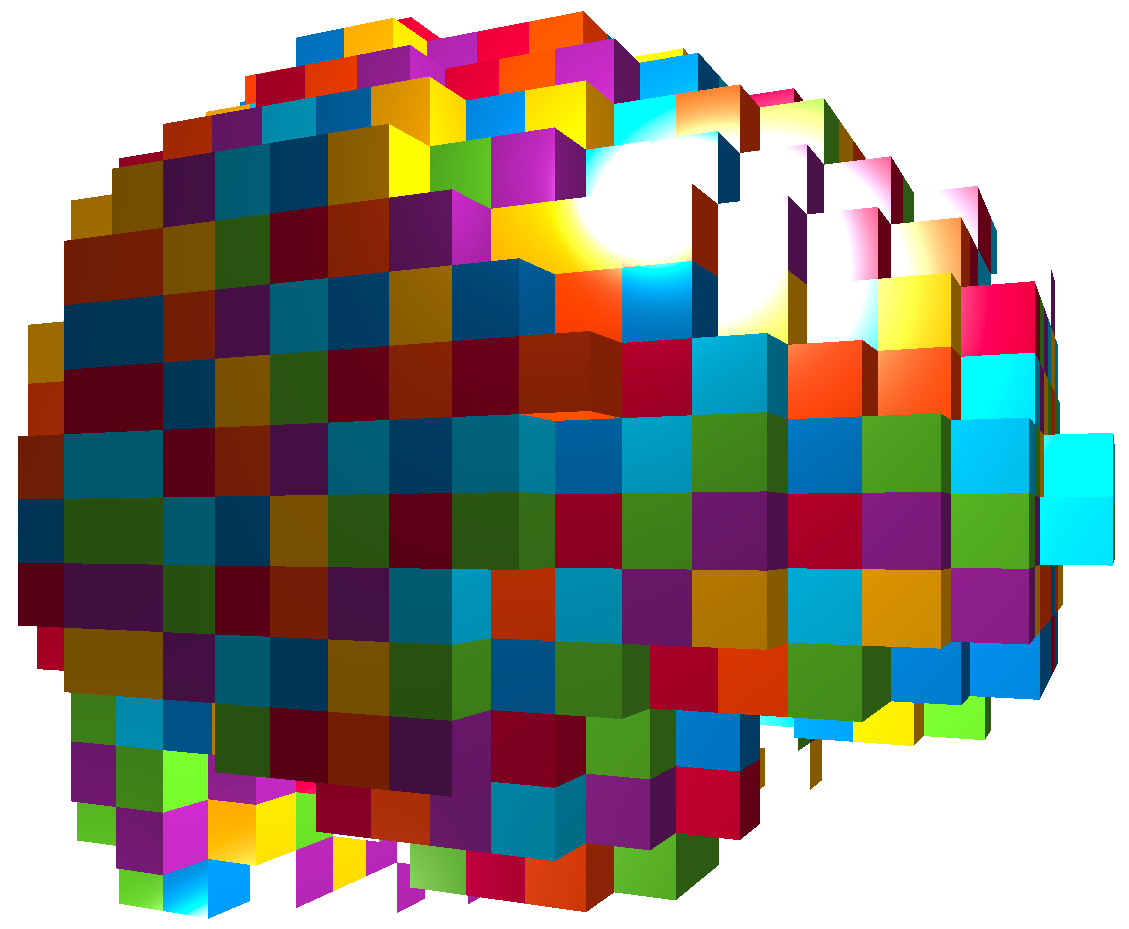Time 12~300

Sub i, Sess jSub ~100, Sess ~4Large, multilevel (subject, sess, voxel) data
$100 \times 4 \times 300 \times 10^6 \approx 100$ billion data points

## Data Matrix

• $X_{n \times d}$: $n$ observations from $d$ voxels
• Functional segregation: voxel clusters (ROIs)
•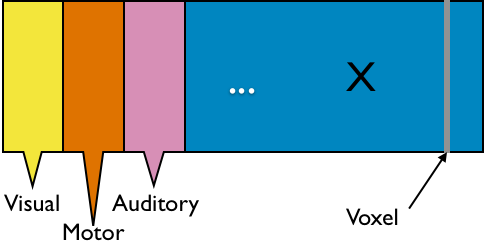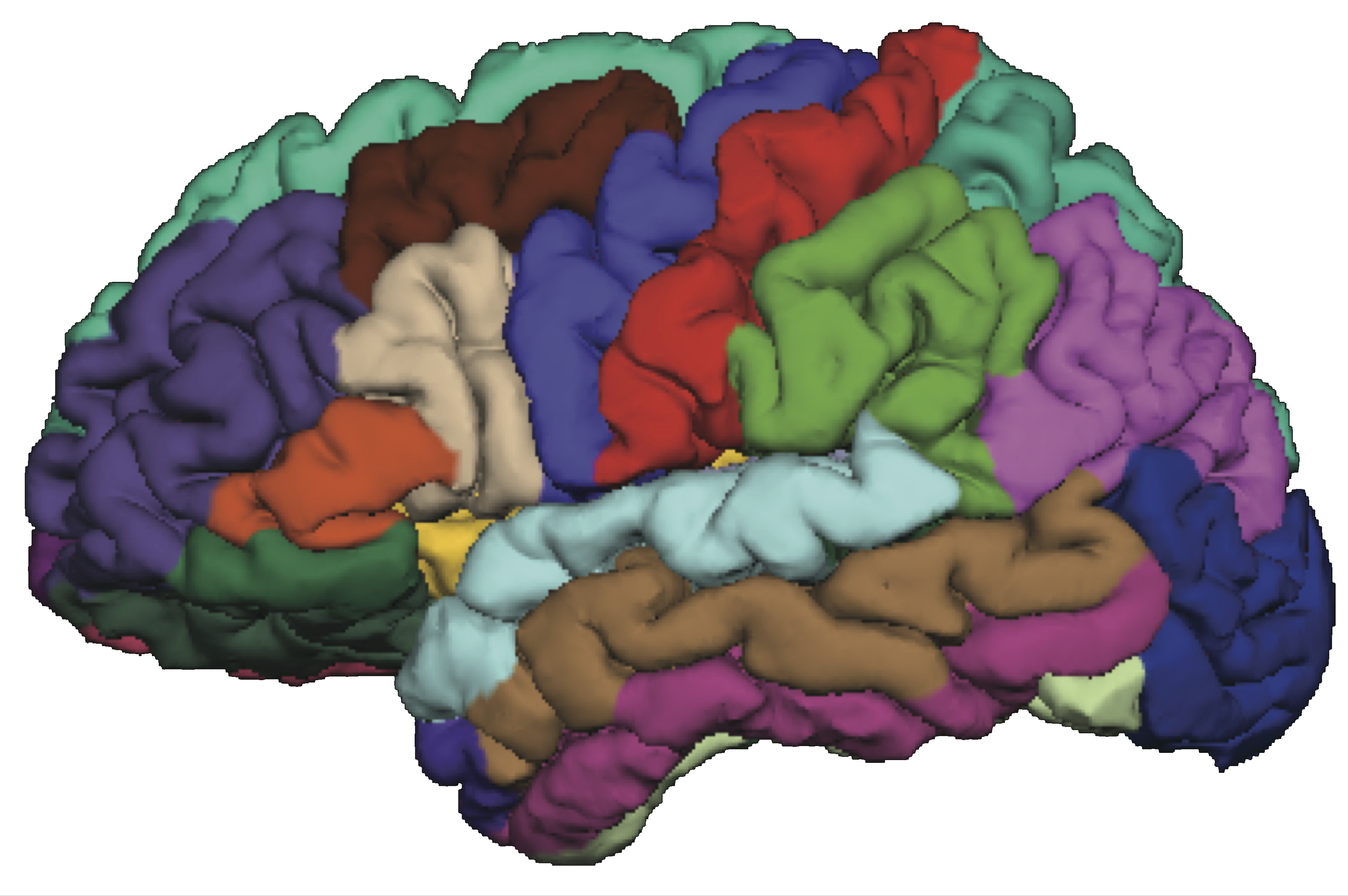• Dimension reduction: $X_{n\times p}$—averages from $p$ ROIs
• Reduced computation: $p \approx 10^2 \ll d \approx 10^6$
• Statistically: introduce bias and reduce efficiency Luo, 14
• Scientifically: ROI definitions can vary greatly
• Still a popular approach due to simplicity (will pursue in this talk)

## Brain Connectivity

• Given $X_{n\times p}$, infer connections between $p$ ROIs.
• Intuition: connected ROIs have similar fluctuations
• Stat objective: infer the direct network connections
• "small n, large p": science, theory, method, computation, and data science
• Temporal dependence removed or small
• Low sampling rate: 300 time points, ~2 seconds apart
• Standard preprocessing (AR models) Lindquist, 06; Bownman, 14
• Causal nonparametric testing Luo et al, JASA 12

# Graphical Models

## Overview

• Data: $n \times p$ matrix of $n$ activities from $p$ voxels
• Methods: biophysical interpretability $\propto$ scalability${}^{-1}$
• Correlation
• PCA, independent component analysis (ICA) Calhoun, Guo, and colleagues
• Graphical models (inverse covariance)
• Granger causality, autoregressive modelsDing, Hu, Ombao, and colleagues
• Bayesian models
• Dynamic models (partial differential equations) Friston and colleagues

## Selected Methods and Opinions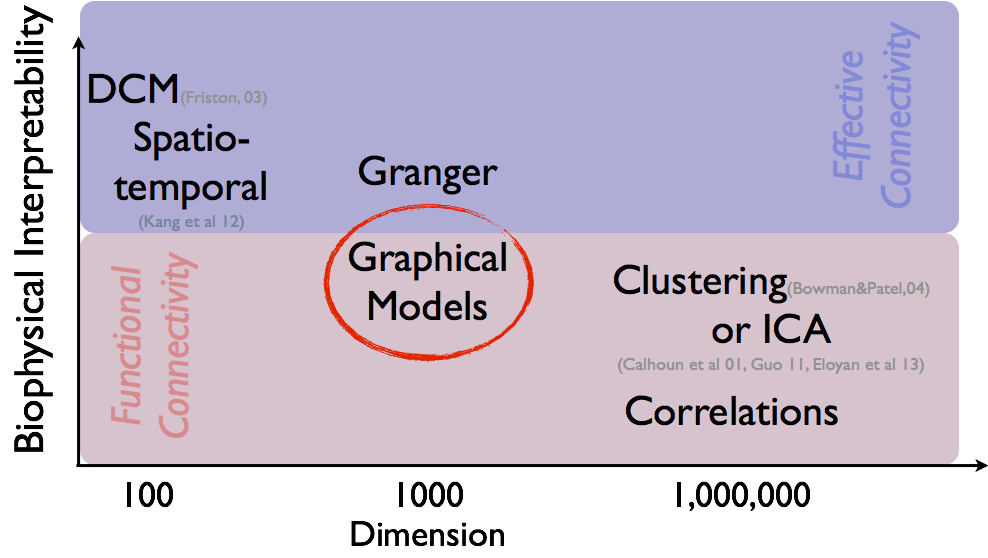## Intro: Graphical Models

• For simplicity, $X\sim^{\textrm{iid}} N(0, \Sigma_{p\times p})$
• All data are centered and scaled usually
• Assumptions can be relaxed (e.g. non-normal data, spatialtemporal correlations), with modifications of our methods
• Interested in estimating $\Omega = \Sigma^{-1}$ because $$\Omega_{ij} = 0 \quad \iff \quad \mbox{variable } i \bot j \, | \, \mbox{others}$$
• Network representation: each variable is a node $$\Omega_{ij} = 0 \quad \iff \quad \mbox{No edge node } i \not \sim j$$

## GM vs Correlation

• Matrix $\Omega$ $\iff$ network:
$$\small \Omega = \begin{pmatrix} 1 & 0.2 & {\color{red} 0} & {\color{red} 0}\\ 0.2 & 1 & -0.1 & 0.1\\ {\color{red} 0} & -0.1 & 1 & 0.3\\ {\color{red} 0} & 0.1 & 0.3 & 1\\ \end{pmatrix}$$

$\iff$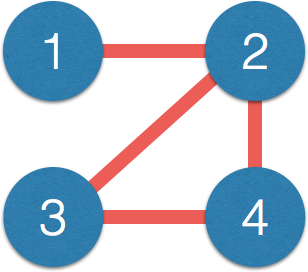• Correlation $\Sigma$ has few zero entries (empirically)
• Path $1-2-3$, $1$ and $3$ correlated but not connected directly
• Correlation measures indirect connections
• Direct connections closer to causal interpretation
• $\Omega$ also related to (known as) partial correlation

## Review: Sparse GM Frameworks

• Sample cov $\hat{\Sigma}$ non-invertible when $p>n$
• Assume sparse $\Omega$: interpretability and stability
• Penalized conditional likelihood Meinshausen & Bulmann, 06
• Penalized (e.g. $\ell_1$ or LASSO) regression: $X_j \sim X_{-j}$
• Colinearity/correlation makes variable selection challenging
• Penalized likelihood using $\hat{\Sigma}$ Yuan & Lin, 07; Banerjee et al, 07; Friedman et al, 08
• Matrix computation, misspecified likelihood
• Slow convergence due to bad global convergence
• Algebraic (non-likelihood) approaches JASA, 11; JMVA 15
• Leveraging good "local"/element-wise convergence

## Algebraic Approach 1: CLIME

• CLIME Cai, Liu, Luo, JASA, 11 $$\min \| \Omega \|_1 \quad \textrm{subject to: } \lVert \hat{\Sigma} \Omega - I \rVert_\infty\le \lambda$$
• $\| \Omega \|_1 = \sum_{ij} \| \Omega_{ij} \|$, $\| M \|_\infty = \max_{i,j} \| M_{ij} \|$.
• Symmetrication: $\Omega = \min(\Omega, \Omega^T)$
• Vector computation: solve columns $\Omega_{\cdot j}$ separately
• Algorithm: linear programming
• Unique solutions, and fast algorithms
• Penalty choice (LASSO) not critical

## Illustration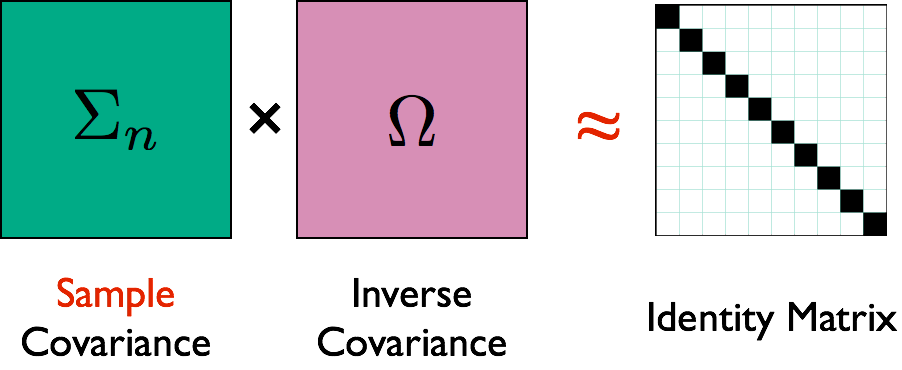## Illustration## Theory

• Assumptions: large $p = O(\exp(n))$ and signal$>\lambda$
• Faster convergence rates than penalized likelihood
• CLIME $n^{-1/2} \log^{1/2} p$ vs likelihood $n^{-1/2} p^{\xi}$ in polynomial-tail dist
• Minimax optimal Cai et al, 14
• Works for semiparametric distributions Liu, Lafferty, Wasserman, and colleagues
• Weaker assumptions than penalized likelihood
• CLIME works under stronger "colinearity"
• Limitations: tuning parameter selection and computation

## Algebraic Approach 2: SCIO

• SCIO Liu, Luo, JMVA, 15 $$\min_{\mathbf{\beta} = \Omega_{\cdot j}}\left\{ \frac{1}{2}\mathbf{\beta}^{T}\hat{\Sigma}\mathbf{\beta}-\mathbf{\beta}^{T}e_{i}+\lambda\left\Vert \mathbf{\beta}\right\Vert _{1}\right\}$$
• Generalization of conjugate gradient loss
• Faster computation via convex programming
• Smaller (constants) than CLIME
• Better rates than penalized likelihood

## New CV and Its Theory

• Random split (training/validating): $n_\mbox{tr} \asymp n_\mbox{val} \asymp n$
• Select tuning $\lambda_i$ on a grid (size $N$) to min loss $$\hat{R}(\lambda_i)= \frac{1}{2}(\hat{\beta}^{\mbox{tr}}(\lambda_i))^{T}\hat{\Sigma}_{\mbox{val}} \hat{\beta}^{\mbox{tr}}(\lambda_i)-e^{T}\hat{\beta}^{\mbox{tr}}(\lambda_i)$$
• Use selected $\hat{\lambda}$ above for SCIO estimator $\hat{\Omega}_{\mbox{cv}}$
Theorem. Under regularity conditions, $\log N = O(\log p)$, $n^{1/2} \log^{-1/2} p = o(N)$, as $n,\,p\rightarrow \infty$, $$\frac{1}{p} \left\| \hat{\Omega}_{\mbox{cv}} - \Omega \right\|_F^2 = O_p \left(\frac{\log p}{n} \right)$$

# Simulations

## Matrix Loss Comparison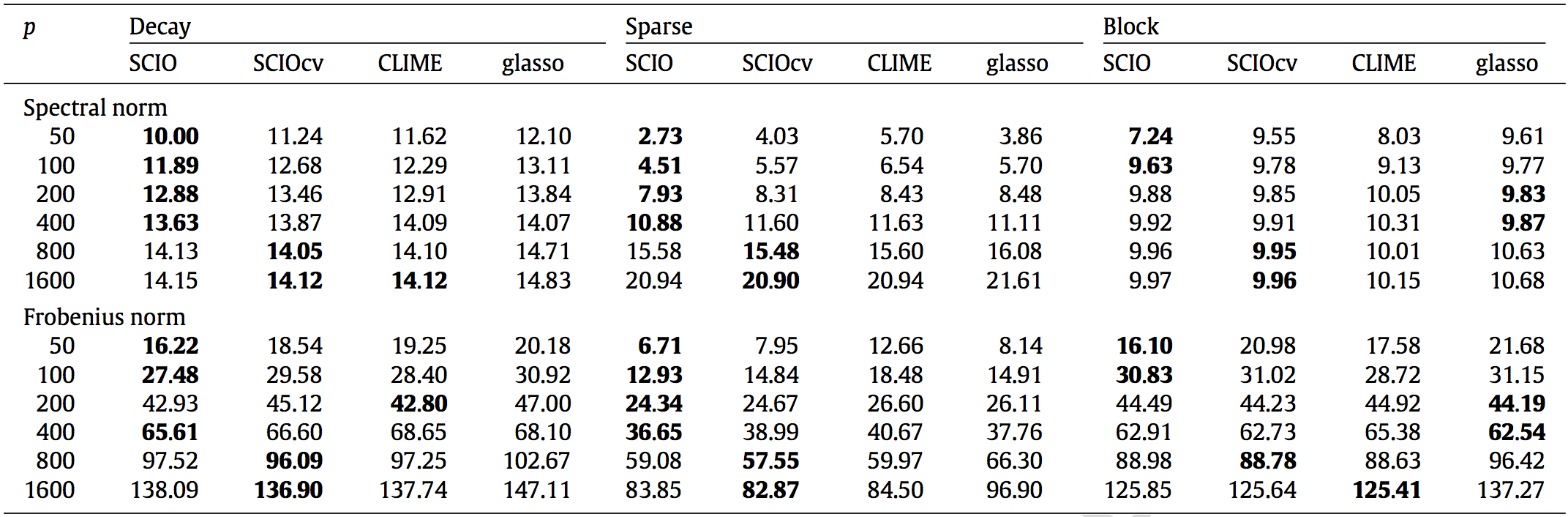SCIO has samller losses for most scenarios

## Network Recovery Comparison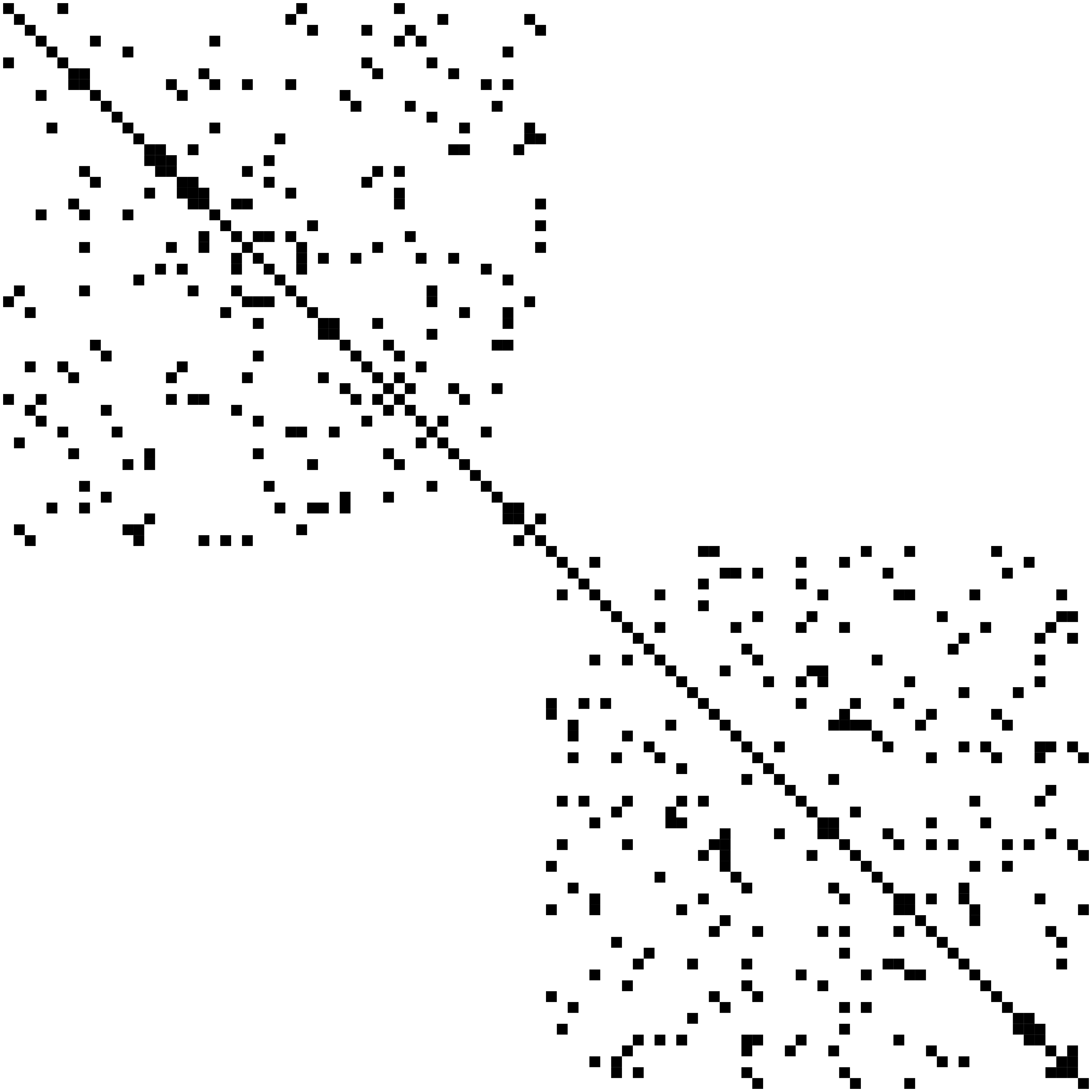Truth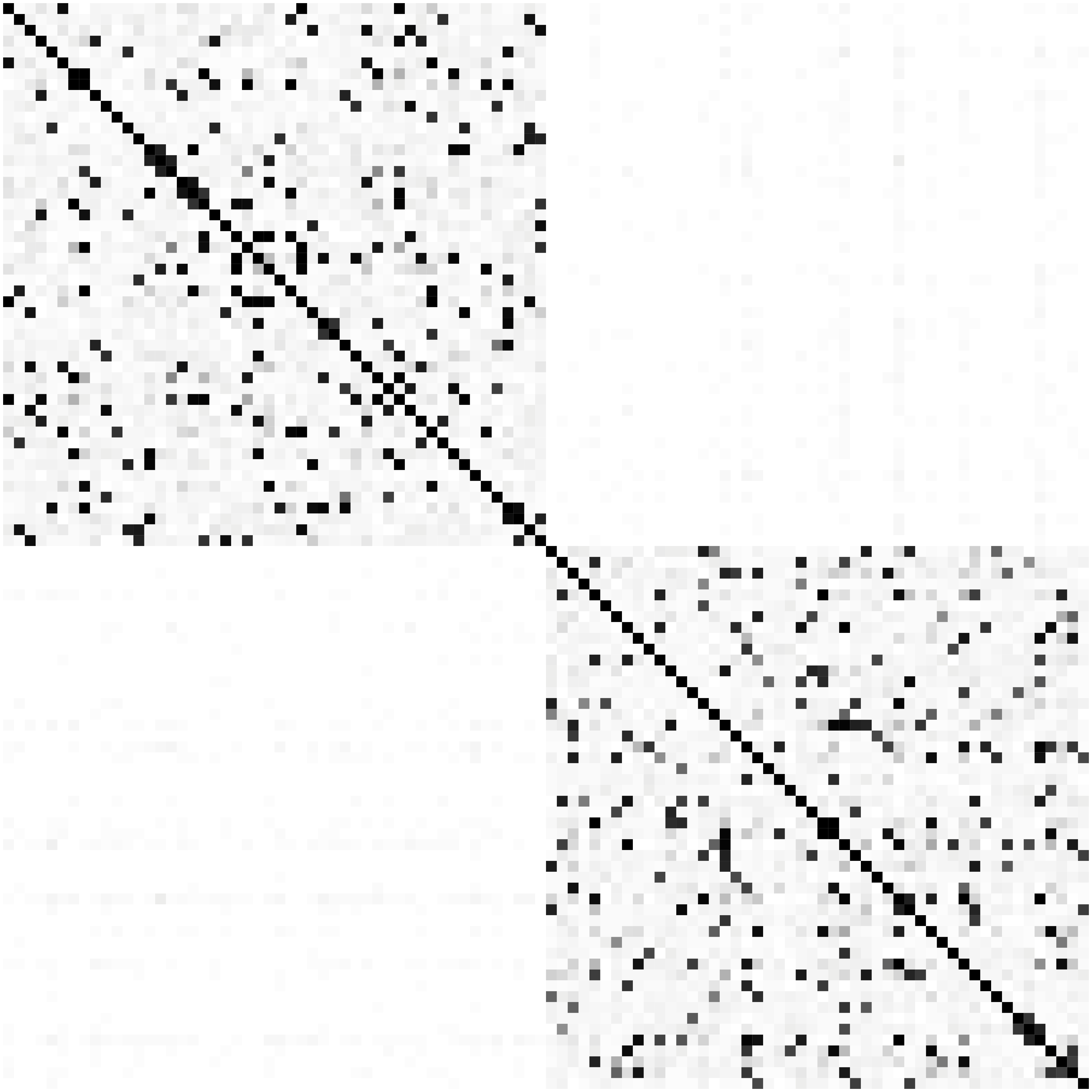SCIO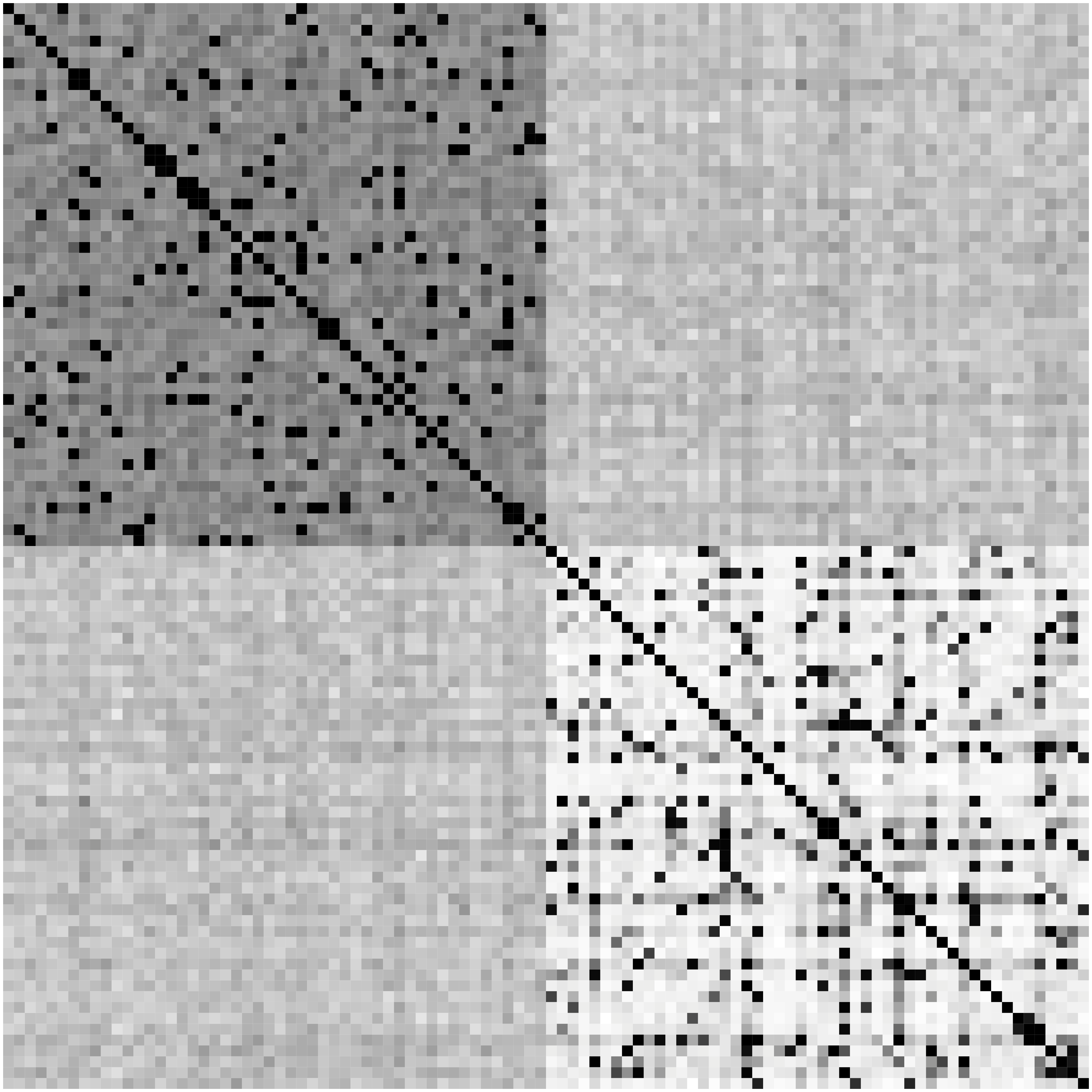Glasso

Heatmaps: black-nonzero over 100% runs; white-100% zero.

SCIO closer to the truth

## fMRI Simulation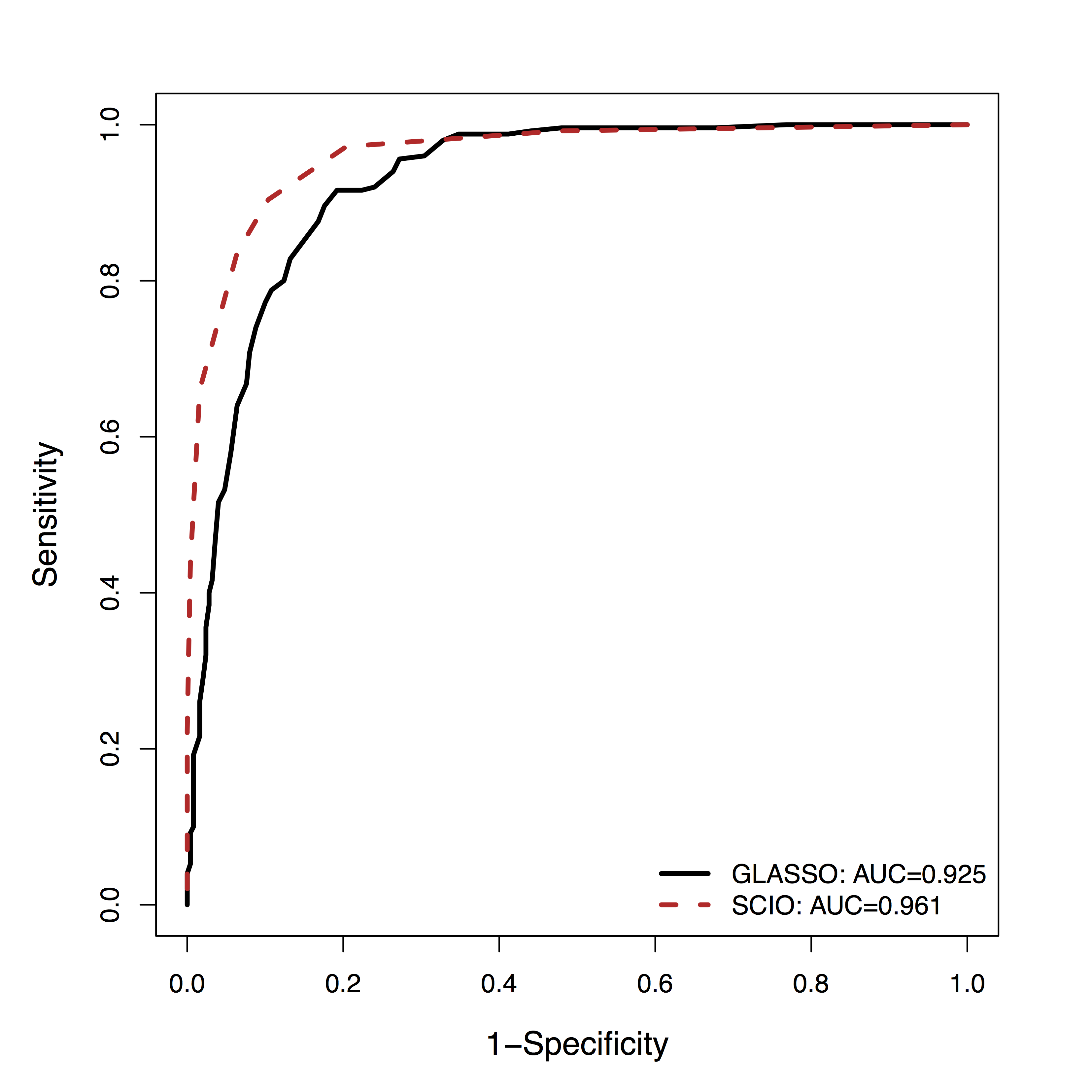• GM is among the top 3 methods of 30+ by massive dynamic simulations (600+ citations) Smith et al, 10
• Using their data, SCIO has better ROC of recovering the connections (non-zero $\Omega$), vs penalized likelihood (GLASSO)

# Real Data

• Data from the ADHD-200 project
• fMRI data from 61 Healthy, 22 ADHD cases
• 116 brain regions (AAL), 148 observationsHeatmaps: black-nonzero over 100% subs; white-100% zero.

SCIO: clearer contrasts between healthy and ADHD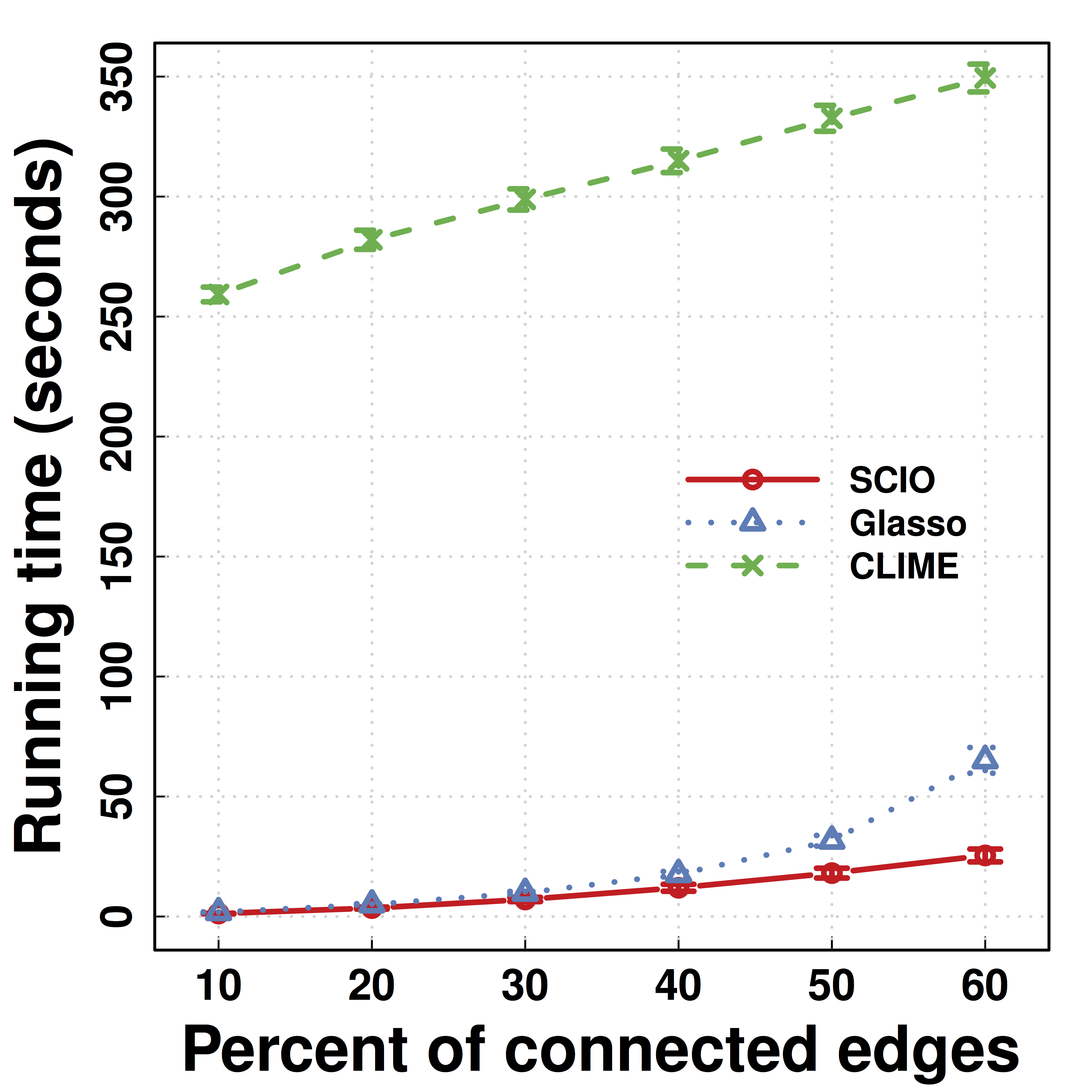SCIO: faster computation and better scaling

## Another Data Example: HIV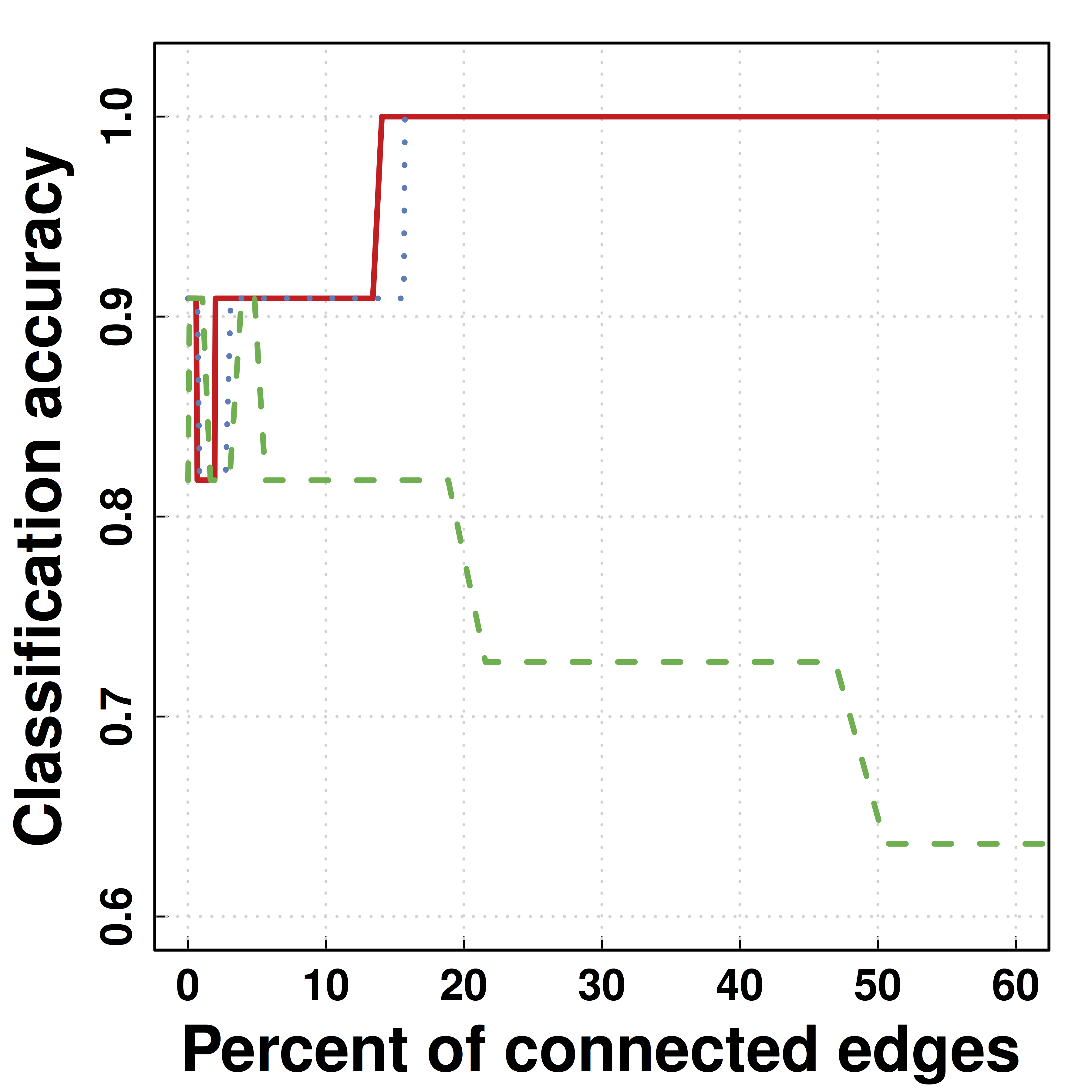• Predicting HIV/non-HIV brains using gene exprs using LDA ($\Omega$)
• SCIO: higher pred accuracy

## Summary

• New loss functions without likelihood
• Improved accuracy and theory
• Fast computation
• Optimization: build methods to recover patterns
• A step for big and complex (network) data
• Accurate network recovery for brain networks
• For a broad range of distributions and data
• Utility: diagnosis and personalized medicine
• Limitations and future work: complex models, faithful dimension reduction, implementation

## CollaboratorsTony Cai
Univ of PennWeidong Liu
Shanghai JiaoTong Univ

## References

• Bunea, Giraud, X Luo. Community Estimation in G-models via CORD. Submitted to Annals Stat
• Luo. A Hierarchical Graphical Model for Big Inverse Covariance Estimation with an Application to fMRI. Revision for Biostat
• Luo, Gee, Sohal, Small (2016). A Point-process Response Model for Optogenetics Experiments on Neural Circuits. Stat Med.
• Liu, Luo (2015). Fast and Adaptive Sparse Precision Matrix Estimation in High Dimensions. J Multivariate Analysis.
• Luo et al (2013) Cognitive control and gender specific neural predictors of relapse in cocaine dependence. Brain
• Luo, Small, Li, Rosenbaum (2012). Inference with Interference between Units in an fMRI Experiment of Motor Inhibition. JASA
• Cai, Liu, Luo (2011). A Constrained $\ell_1$ Minimization Approach to Sparse Precision Matrix Estimation. JASA
• R packages: clime, cord, pro, scio